几何变换_几何变换详解 - CSDN

• 一、基本的二维几何变换1.二维变换通过将位移量加到一个点的坐标上来生成一个新的坐标位置，可以实现一次平移（translation）。 下面的程序演示了平移操作。输入的平移向量用来将一个多边形的n个顶点从一个世界...
一、基本的二维几何变换

1.二维变换

通过将位移量加到一个点的坐标上来生成一个新的坐标位置，可以实现一次平移（translation）。
下面的程序演示了平移操作。输入的平移向量用来将一个多边形的n个顶点从一个世界坐标系移动到另一个，而OpenGl子程序用来重新生成平移后的多边形。

class wcPt2D
{
public:
GLfloat x, y;
};
void translatePolygon(wcPt2D *verts, GLint nVerts, GLfloat tx, GLfloat ty)
{
GLint k;
for (k = 0; k < nVerts; k++)
{
verts[k].x = verts[k].x + tx;
verts[k].y = verts[k].y + ty;
}
glBegin(GL_POLYGON);
for (k = 0; k < nVerts; k++)
glVertex2f(verts[k].x, verts[k].y);
glEnd();
}


如果我们要删除原来的多边形则可以在平移前用背景色显示它。同样，如果我们要保存原来的多边形位置，可以将平移后的多边形存入不同的数组。
可以使用同样的方法来平移其他对象，例如，对于圆或者椭圆，我们可以平移中心坐标并在新的中心坐标上重画图形。对于曲线，通过平移定义该曲线路径的点，然后使用平移过的坐标位置来重构曲线。

2.二维旋转

通过指定一个旋转轴（rotation axis）和一个旋转角度（rotation angle），可以进行一次旋转（rotation）变换。
类似平移，旋转是一种不变形的刚体变换，对象上的所有点旋转相同角度。线段的旋转可以通过将下列旋转方程用于每个线段端点，并重新绘制新端点间的线段而得到。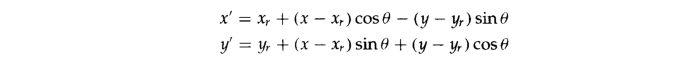多边形的旋转则是将每个顶点旋转指定的旋转角，并使用新的顶点来生成多边形而实现旋转。曲线的旋转通过定位定义的点并重新绘制曲线而完成。例如圆或椭圆，可以通过将中心位置沿指定旋转角对着的弧移动而绕非中心轴旋转。
椭圆可以通过旋转其长轴和短轴来实现绕其中心位置的旋转。
在下列程序示列中，一个多边形绕指定的世界坐标系中的基准点旋转。旋转过程的输入参数是原始的多边形顶点，基准点坐标和用弧度表示的旋转角θ，多边形用OpenGL子程序重新生成。

 class wcPt2D
{
public:
GLfloat x, y;
};                              void   rotatePolygon(wcPt2D*verts,GLint nverts,wcPt2D pivPt,GLdouble theta)
{
wcPt2D * vertsRot;
GLint k;
for (k = 0; k < nVerts; k++)
{
vertsRot[k].x = pivPt.x + (verts[k].x - pivPt.x)*cos(thrta) - (verts[k].y - pivPt.y)*sin(theta);
vertsRot[k].y= pivPt.y + (verts[k].x - pivPt.x)*sin(thrta) + (verts[k].y - pivPt.y)*cos(theta);

}
glBegin(GL_POLYGON);
for (k = 0; k < nVerts; k++)
glVertex2f(vertsRot[k].x, vertsRot[k].y);
glEnd();
}


3.二维缩放

改变一个对象大小，可使用缩放（scaling）变换。
多边形的缩放可以通过将以下变换方程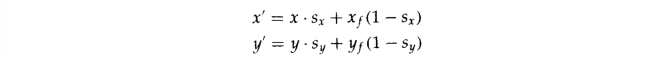应用于每个顶点，然后利用变换后的顶点重新生成多边形而实现。要改变圆的大小，可通过缩放其半径并计算圆上坐标点的新坐标位置来实现。标准位置中的椭圆通过缩放两个轴并按其中心坐标重新绘制椭圆而实现缩放。
下列程序，多边形顶点和固定点的坐标以及缩放系数是输入参数。坐标变换后，使用OpenGL子程序重新生成缩放后的多边形。

class wcPt2D
{
public:
GLfloat x, y;
};
void sacalPolygon(wcPt2D*verts,GLint nverts,wcPt2D fixedPt,GLfloat sx,GLfloat sy)
{
wcPt2D  vertsNew;
GLint k;
for (k = 0; k < nVerts; k++)
{
vertsNew[k].x = verts[k].x*sx + fixedPt.x*(1 - sx);
vertsNew[k].y = verts[k].y*sy+fixedPt.y*(1-sy);
}
glBegin(GL_POLYGON);
for (k = 0; k < nVerts; k++)
glVertex2f(vertsNew[k].x, vertsNew[k].y);
glEnd();
}

展开全文• ## 图像几何变换

千次阅读 2020-04-19 11:43:48
一，求点(x1, y1)关于点(x0, y0)旋转a度后的坐标 1、首先可以将问题简化，先算点(x1, y1)关于源点逆时针旋转a度后的坐标，求出之后加上x0，y0即可。 2、关于源点旋转，用极坐标表示 设x1 = Rcos(θ), y1 = Rsin...
一.仿射变换概念

1.下图是一般形式，其中x,y代表原坐标，v,w代表变换后的坐标，T是变换矩阵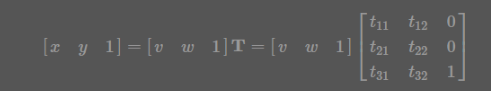其中几种常见的变换形式矩阵为：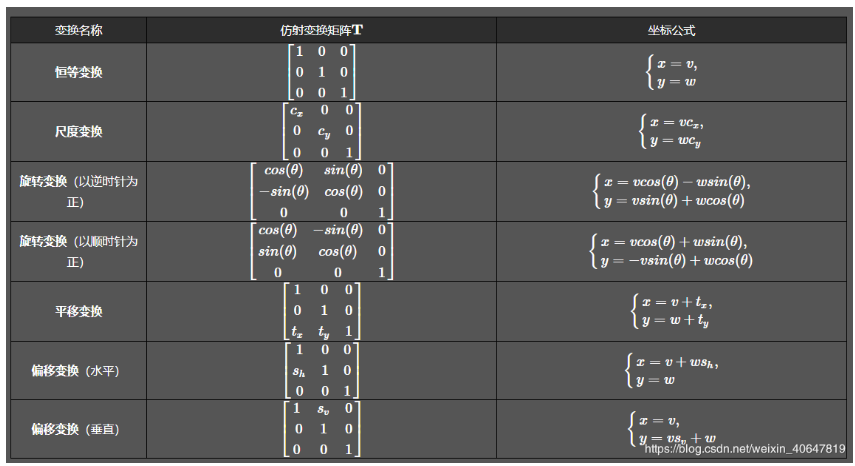2.坐标系变换

再看第二个问题，变换中心，对于缩放、平移可以以图像坐标原点（图像左上角为原点）为中心变换，这不用坐标系变换，直接按照一般形式计算即可。而对于旋转和偏移，一般是以图像中心为原点，那么这就涉及坐标系转换了。

我们都知道，opencv的原点在图像左上角，水平向右为 X 轴，垂直向下为 Y 轴。课本中常见的坐标系是以图像中心为原点，水平向右为 X 轴，垂直向上为 Y 轴，称为笛卡尔坐标系。看下图: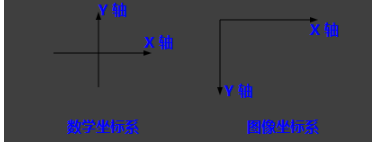因此，对于旋转和偏移，就需要3步（3次变换）：

将输入原图图像坐标转换为笛卡尔坐标系；
进行顺时针旋转计算。旋转矩阵前面已经给出了；
将旋转后的图像的笛卡尔坐标转回图像坐标。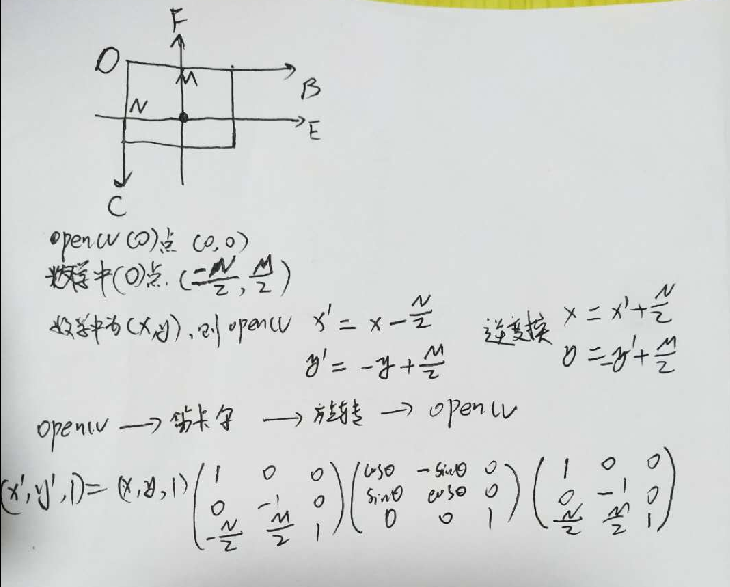3.示例：（250,0）绕（250,250）旋转120度

import cv2
import numpy as np
from math import cos,sin,pi

def rotate_one(x,y,angle,cx,cy):

"""
点(x,y) 绕(cx,cy)点顺时针旋转
"""
angle = angle*pi/180
x_new = (x-cx)*cos(angle) + (cy-y)*sin(angle)+cx
y_new = (x-cx)*sin(angle) + (y-cy)*cos(angle)+cy
return x_new, y_new

def rotate_two(x,y,angle,cx,cy):
"""
点(x,y) 绕(cx,cy)点顺时针旋转
"""
angle = angle * pi / 180
input_matrix = np.array([x, y, 1])
transform_matrix = np.array([[1, 0, 0],
[0, -1, 0],
[-cx, cy, 1]])
inv_transform_matrix = np.array([[1, 0, 0],
[0, -1, 0],
[cx, cy, 1]])
rotate_matrix = np.array([[cos(angle), -sin(angle), 0],
[sin(angle), cos(angle), 0],
[0,           0,         1]])
output_matrix = ((input_matrix.dot(transform_matrix)).dot(rotate_matrix)).dot(inv_transform_matrix)
x_new, y_new, _ = output_matrix
return x_new, y_new
def test_cv2():

img=np.zeros((501,501))

x, y= 250, 0
cx,cy=250,250
angle=120
# x_new, y_new = rotate_one(x,y,angle,cx,cy)
# cv2.circle(img, (cx, cy), radius=2, color=(255, 255, 255), thickness=2)
# cv2.circle(img, (int(x_new), int(y_new)), radius=2, color=(255, 255, 255), thickness=2)
# cv2.imshow('img', img)
# cv2.waitKey(0)

x_new, y_new = rotate_two(x,y,angle,cx,cy)
cv2.circle(img, (cx, cy), radius=2, color=(255, 255, 255), thickness=2)
cv2.circle(img, (int(x_new), int(y_new)), radius=2, color=(255, 255, 255), thickness=2)
cv2.imshow('img', img)
cv2.waitKey(0)

if __name__ == '__main__':
test_cv2()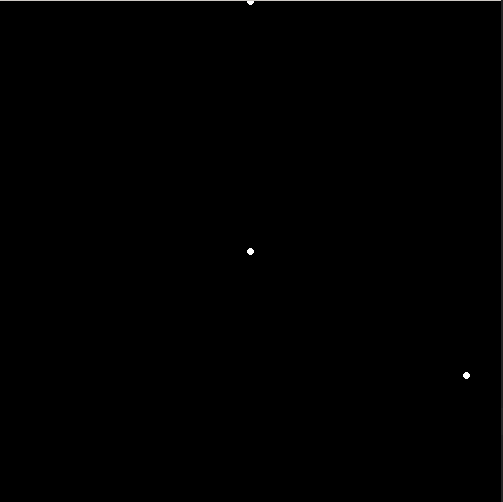4.代码示例：对图中的最大轮廓猪猪旋转180度

import cv2
import numpy as np
from math import cos,sin,pi
import imutils
from matplotlib import pyplot as plt

def rotate(points, angle, cx, cy):
"""
点(x,y) 绕(cx,cy)点顺时针旋转
"""
h, w = points.shape
one = np.ones((h, 1))
input_matrix = np.hstack((points,one))
print(input_matrix.shape)
print(input_matrix[:2])
angle = angle * pi / 180

# input_matrix = np.array([x, y, 1])
transform_matrix = np.array([[1, 0, 0],
[0, -1, 0],
[-cx, cy, 1]])
inv_transform_matrix = np.array([[1, 0, 0],
[0, -1, 0],
[cx, cy, 1]])
rotate_matrix = np.array([[cos(angle), -sin(angle), 0],
[sin(angle), cos(angle), 0],
[0,           0,         1]])
output_matrix = ((input_matrix.dot(transform_matrix)).dot(rotate_matrix)).dot(inv_transform_matrix).astype(np.int)
# print(output_matrix.shape)
# print(output_matrix[:2])
# x_new, y_new, _ = output_matrix
return output_matrix[:, :-1]

def test_pig_rotate():
path = './20181011234118419.jpeg'
gary = cv2.cvtColor(img,cv2.COLOR_BGR2GRAY)
black=np.zeros([img.shape, img.shape])
#二值化找轮廓
image_thre = cv2.threshold(gary, 127, 255, cv2.THRESH_BINARY)
cnts = cv2.findContours(image_thre, cv2.RETR_EXTERNAL, cv2.CHAIN_APPROX_SIMPLE)
contours = cnts if imutils.is_cv2() else cnts
c_ = sorted(contours, key=cv2.contourArea, reverse=True)
points = np.squeeze(c_)

# #debug show
plt.figure(figsize=(15,15))
# plt.plot(points[:, 0], points[:, 1])
# plt.show()

contour_list = []
new_points = rotate(points, angle=120, cx=img.shape//2, cy=img.shape//2)
contour_list.append(new_points[:, np.newaxis, :])

black = cv2.drawContours(black, contour_list, -1, (255, 255, 255), thickness=-1)
cv2.imwrite('./3.jpg', black)

if __name__ == '__main__':
# test_cv2()
test_pig_rotate()

原图：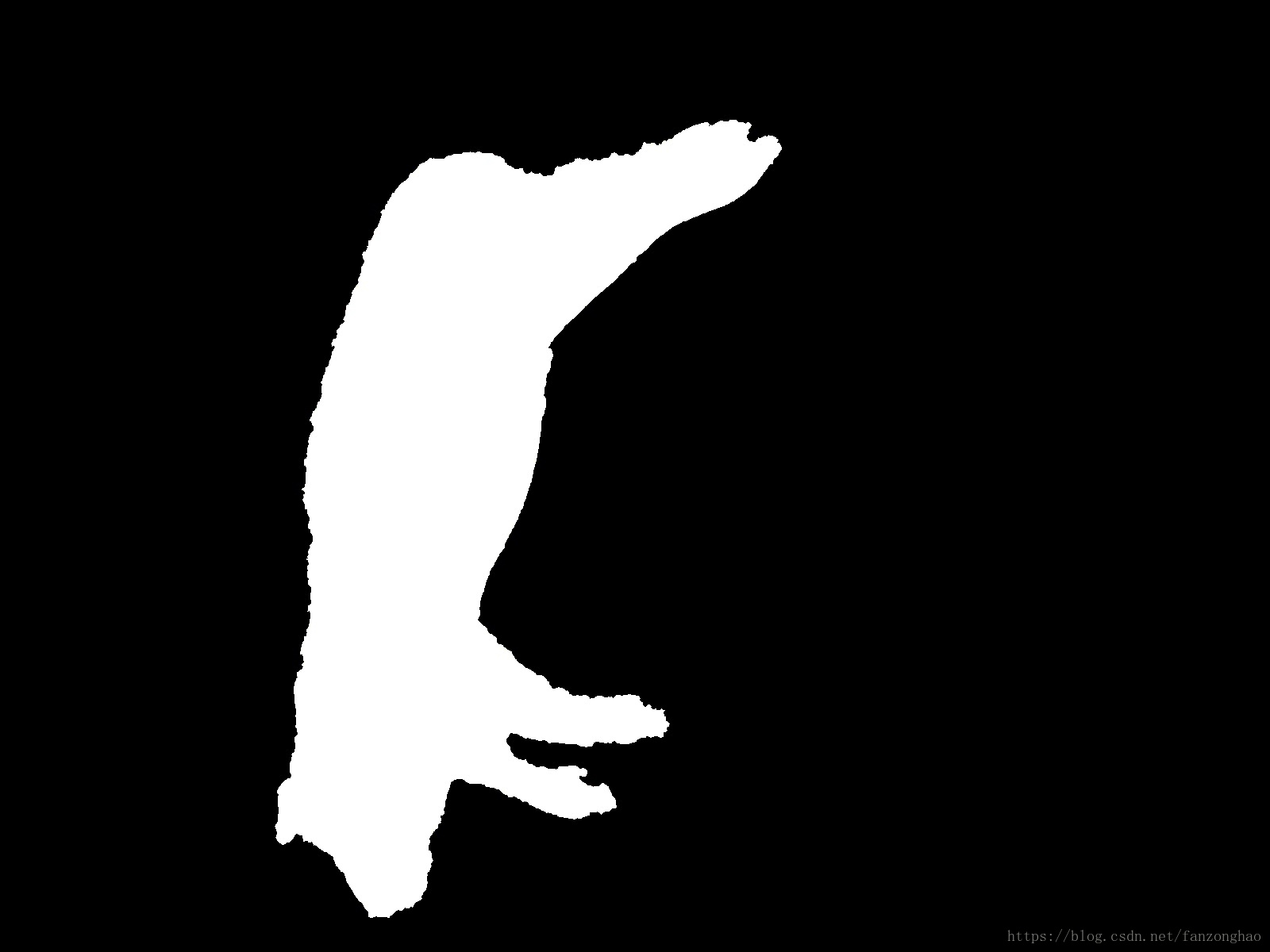顺时针旋转180度：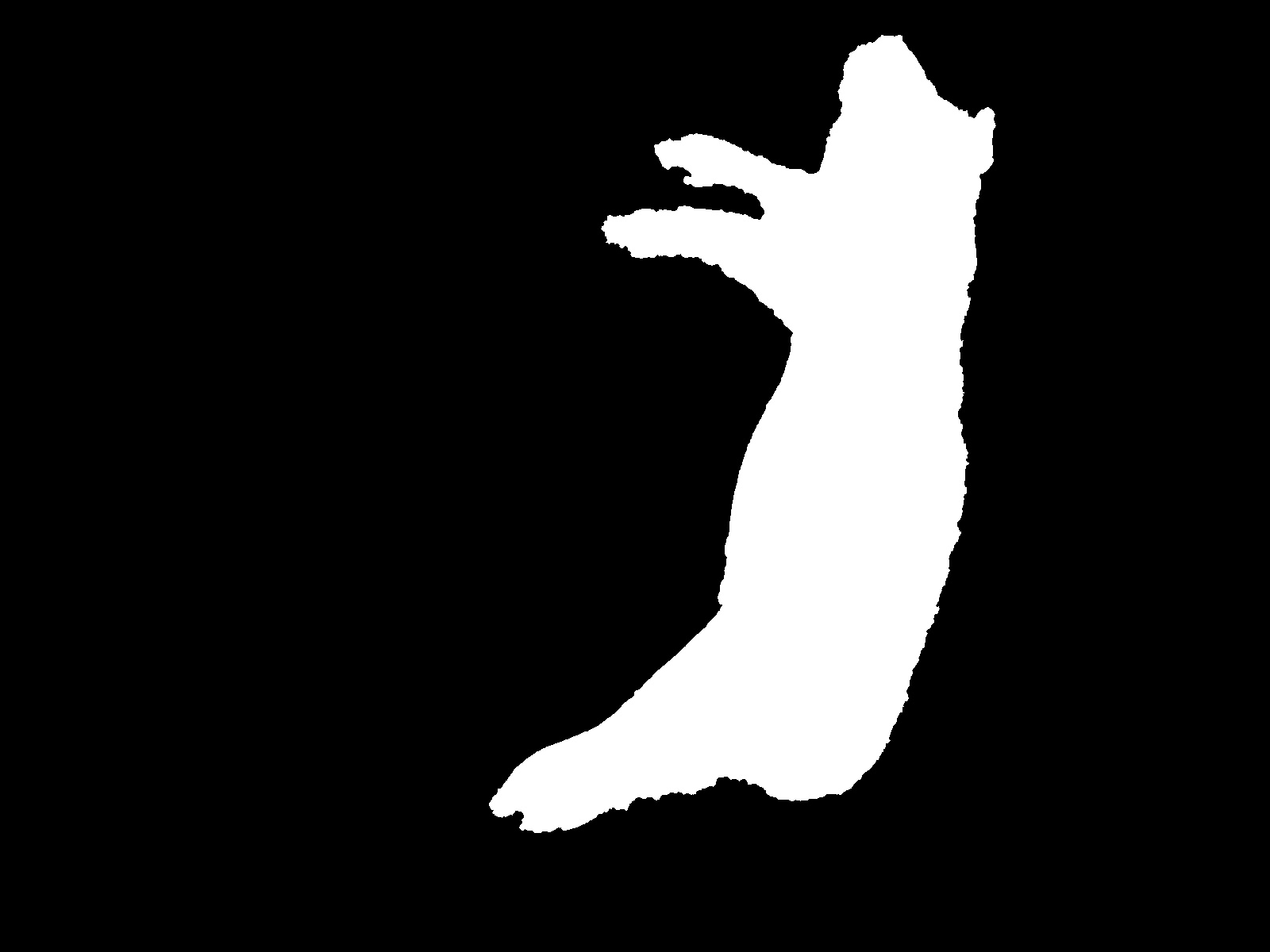5.代码示例：对整张图进行旋转

# 旋转图片无黑边
def rotate_image():
from math import fabs
from math import sin, cos, radians
path = './20181011234118419.jpeg'
height, width = img.shape[:2]

degree = 90
# 旋转后的尺寸

matRotation = cv2.getRotationMatrix2D((width / 2, height / 2), degree, 1)

# print(matRotation[0, 2])
# print(matRotation)
matRotation[0, 2] += (widthNew - width) / 2
matRotation[1, 2] += (heightNew - height) / 2

imgRotation = cv2.warpAffine(img, matRotation, (widthNew, heightNew), borderValue=(255, 255, 255))
#
# cv2.imshow('img', imgRotation)
# cv2.waitKey(0)
cv2.imwrite('img_size_1_ok.jpg', imgRotation)
if __name__ == '__main__':
rotate_image()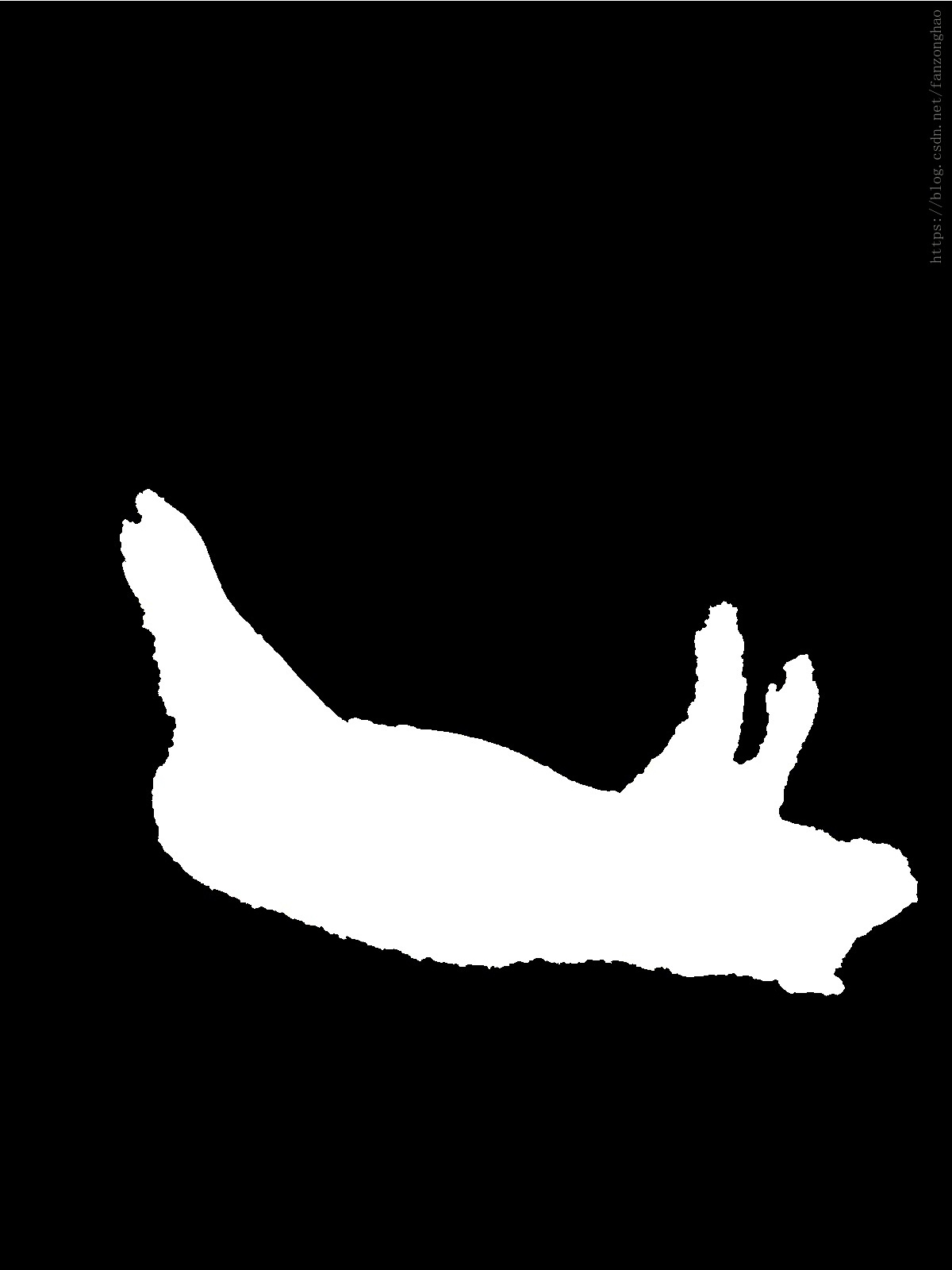展开全文图像旋转
• ## 几何变换详解

千次阅读 2018-08-22 21:41:59
在三维图形学中，几何变换大致分为三种，平移变换（Translation），缩放变换（Scaling），旋转变换（Rotation）。以下讨论皆针对DirectX，所以使用左手坐标系。 平移变换 将三维
原文地址为：几何变换详解在三维图形学中，几何变换大致分为三种，平移变换（Translation），缩放变换（Scaling），旋转变换（Rotation）。以下讨论皆针对DirectX，所以使用左手坐标系。
平移变换
将三维空间中的一个点[x, y, z, 1]移动到另外一个点[x', y', z', 1]，三个坐标轴的移动分量分别为dx=Tx, dy=Ty, dz=Tz, 即
x' = x + Tx
y' = y + Ty
z' = z + Tz
平移变换的矩阵如下。缩放变换
将模型放大或者缩小，本质也是对模型上每个顶点进行放大和缩小（顶点坐标值变大或变小），假设变换前的点是[x, y, z, 1]，变换后的点是[x', y', z', 1]，那么
x' = x * Sx
y' = y * Sy
z' = z * Sz
缩放变换的矩阵如下。旋转变换
这是三种变换中最复杂的变换，这里只讨论最简单的情况，绕坐标轴旋转，关于绕任意轴旋转，在后续的随笔中介绍。
绕X轴旋转绕X轴旋转时，顶点的x坐标不发生变化，y坐标和z坐标绕X轴旋转θ度，旋转的正方向为顺时针方向（沿着旋转轴负方向向原点看）。[x, y, z, 1]表示变换前的点，[x', y', z', 1]表示变换后的点。变换矩阵如下。
关于旋转的正方向，OpenGL与多数图形学书籍规定旋转正方向为逆时针方向（沿着坐标轴负方向向原点看），比如Computer Graphics C Version，p409。绕Y轴旋转绕Y轴旋转时，顶点的y坐标不发生变化，x坐标和z坐标绕Y轴旋转θ度。[x, y, z, 1]表示变换前的点，[x', y', z', 1]表示变换后的点。变换矩阵如下。绕Z轴旋转绕Z轴旋转时，顶点的z坐标不发生变化，x坐标和y坐标绕Z轴旋转θ度。[x, y, z, 1]表示变换前的点，[x', y', z', 1]表示变换后的点。变换矩阵如下。绕坐标轴旋转的矩阵推导
上面三个旋转矩阵是如何得来的呢？我们推导一下，首先看一下二维的情况，再扩展到三维即可。实际上上面三种绕坐标轴旋转的情况属于特殊的二维旋转，比如绕Z轴旋转，相当于在与XOY平面上绕原点做二维旋转。
假设点P(x, y)是平面直角坐标系内一点，其到原点的距离为r，其与X轴的夹角为A，现将点P绕原点旋转θ度，得到点P'(x', y')，P'与X轴的夹角为B，则A = B - θ。（注意，在二维坐标中，逆时针旋转时角度为正，顺时针旋转时角度为负，下图中由P旋转到P'，角度为θ，若是由P'转到P，则角度为-θ）。于是可得下面的转换方程（式一）
写成矩阵的形式就是求得旋转矩阵为由于这里使用齐次坐标，所以还需加上一维，最终变成如下形式
绕Z轴旋转矩阵和前面给出的绕Z轴旋转矩阵完全吻合。
对于绕X轴旋转的情况，我们只需将式一中的x用y替换，y用z替换，z用x替换即可。替换后得到(式二)
对应的旋转矩阵为
绕X轴旋转矩阵对于绕Y轴旋转的情况，只需对式二做一次同样的替换即可，的到的变换方程为对应的变换矩阵为
绕Y轴旋转矩阵逆矩阵
平移变换矩阵的逆矩阵与原来的平移量相同，但是方向相反。旋转变换矩阵的逆矩阵与原来的旋转轴相同但是角度相反。缩放变换的逆矩阵正好和原来的效果相反，如果原来是放大，则逆矩阵是缩小，如果原来是缩小，则逆矩阵是放大。== Happy Coding ==转载请注明本文地址：几何变换详解
展开全文详解
• 图形变换：是一种几何变换，在二维图形处理过程中，常常需要对平面图形的形状，尺寸，显示方向和显示位置进行修改，来达到改变图形的目的。 几何变换：是一种先行变换，对原来图形中的一点坐标通过变换生成一个新的...
几何变化

一、概述

图形变换：是一种几何变换，在二维图形处理过程中，常常需要对平面图形的形状，尺寸，显示方向和显示位置进行修改，来达到改变图形的目的。

几何变换：是一种先行变换，对原来图形中的一点坐标通过变换生成一个新的点坐标：对原来图形中的一条直线的变换是通过直线上的亮点作变换后的新的端点坐标，然后连接这两个新的端点，便得到变换后的直线；类似的，可以进行各种图形的几何变换。几何变换的表示采用3*3矩阵的形式，称为变换矩阵，点的坐标表示采用齐次坐标形式（齐次坐标就是将一个原本是n维的向量用一个n+1维向量来表示，是指一个用于投影几何里的坐标系统，如同用于欧氏几何里的笛卡儿坐标一般。），故几何变换操作的过程是将变换矩阵M作用于齐次坐标点P生成新的坐标点P‘，P’=PM，下边讨论4中基本坐标变换。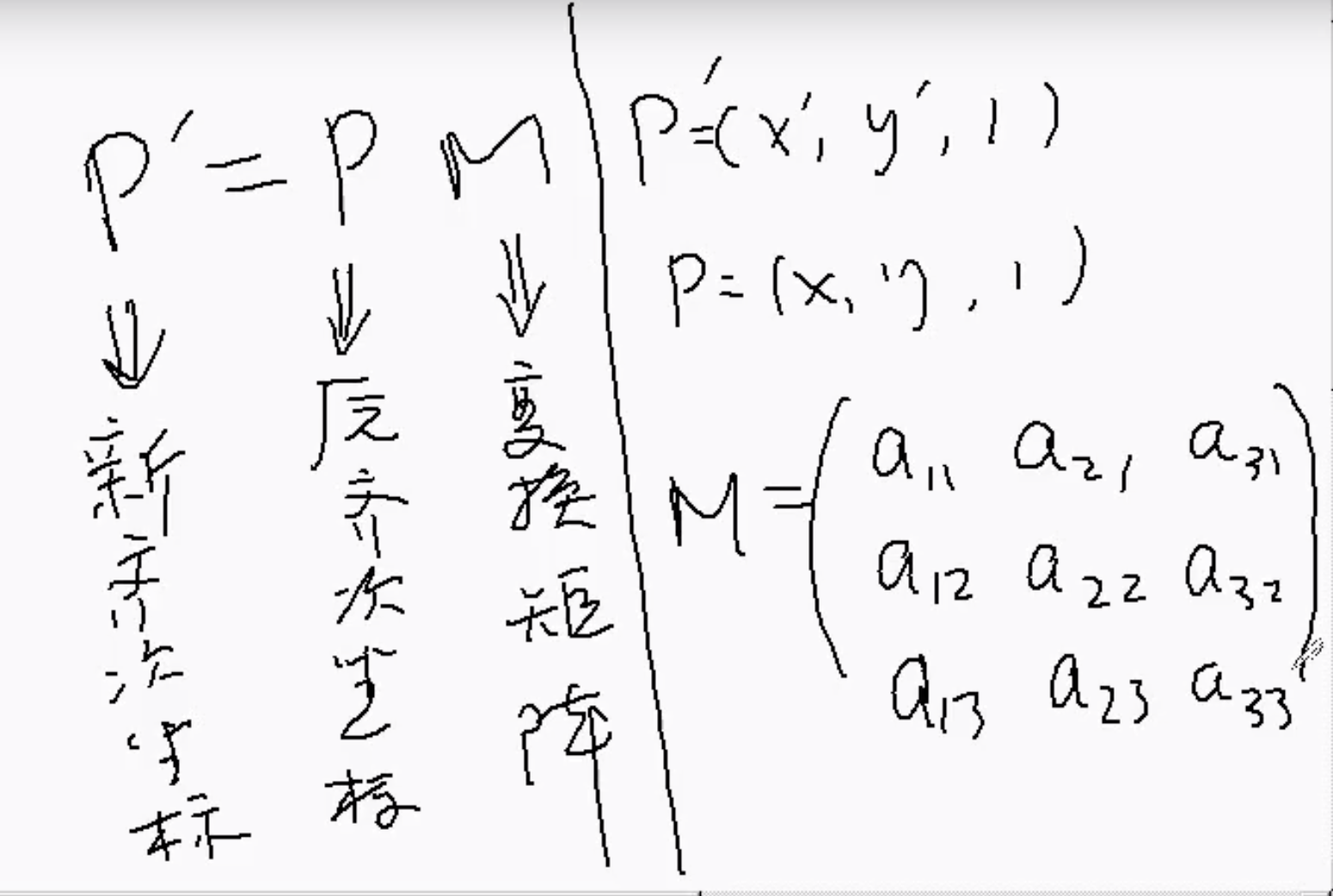二、平移变换

点的平移变换是指该点在X轴和Y轴方向上分别移动一段距离。设图形上点P(x,y)，将x轴和y轴方向分别移动Tx和Ty，结果生成新的点P‘(x',y'),如图所示则有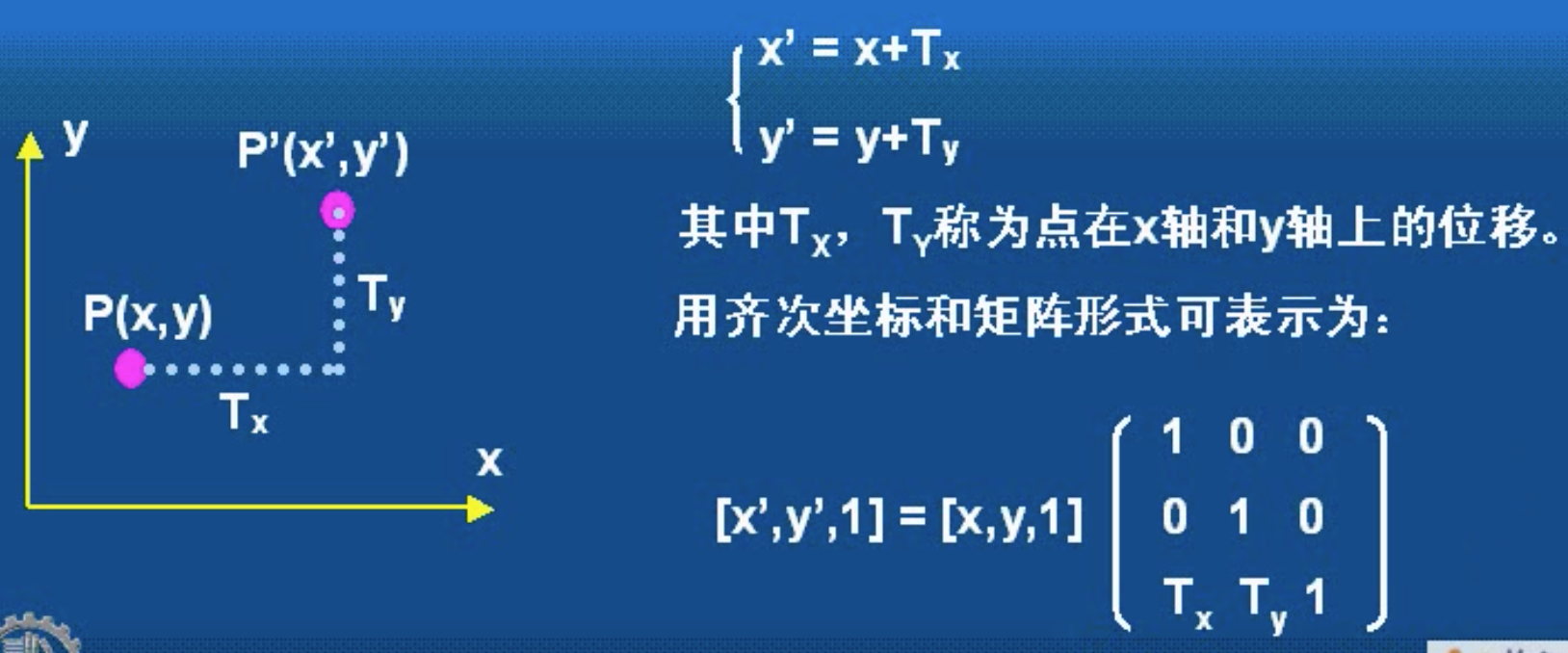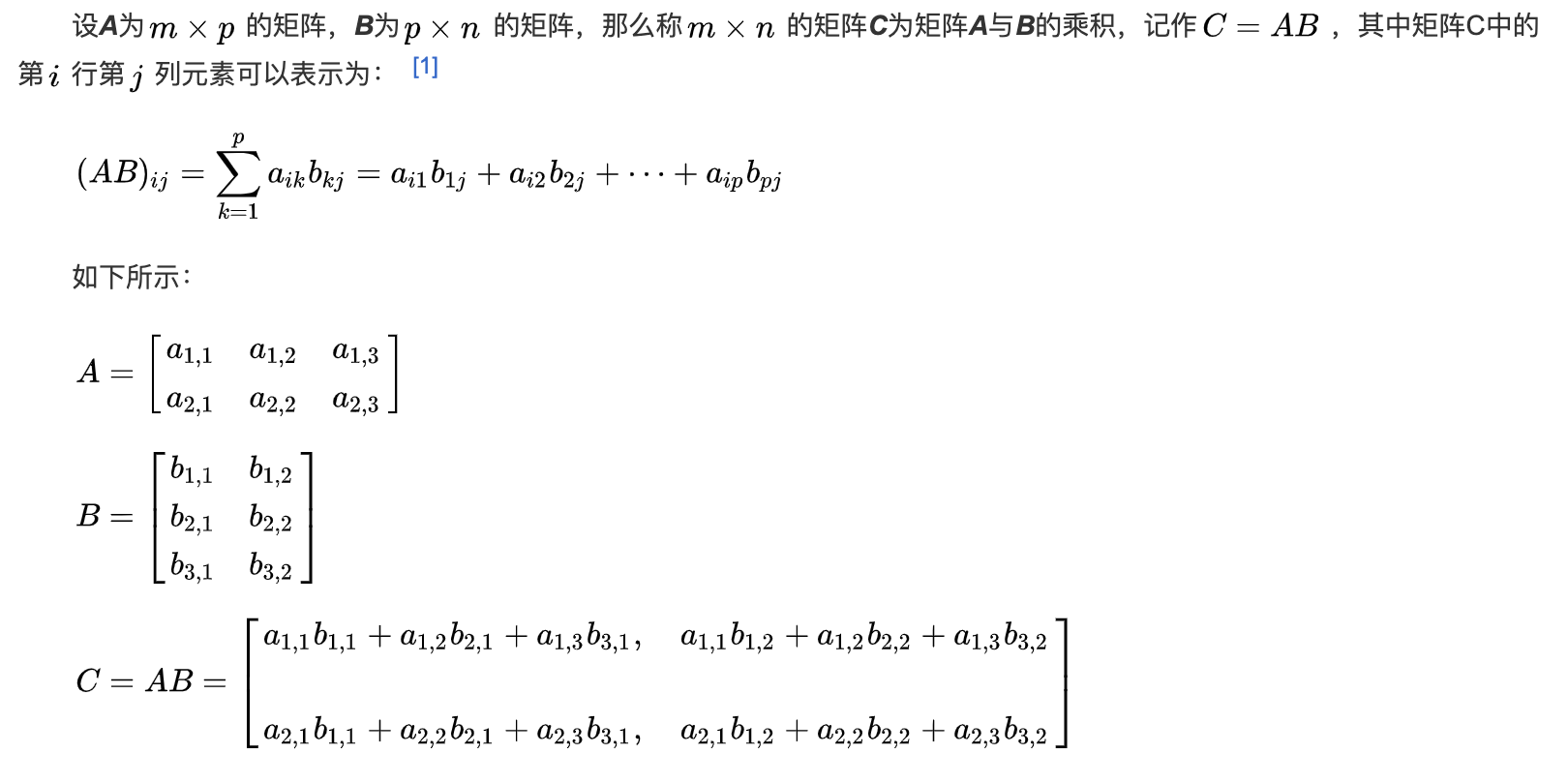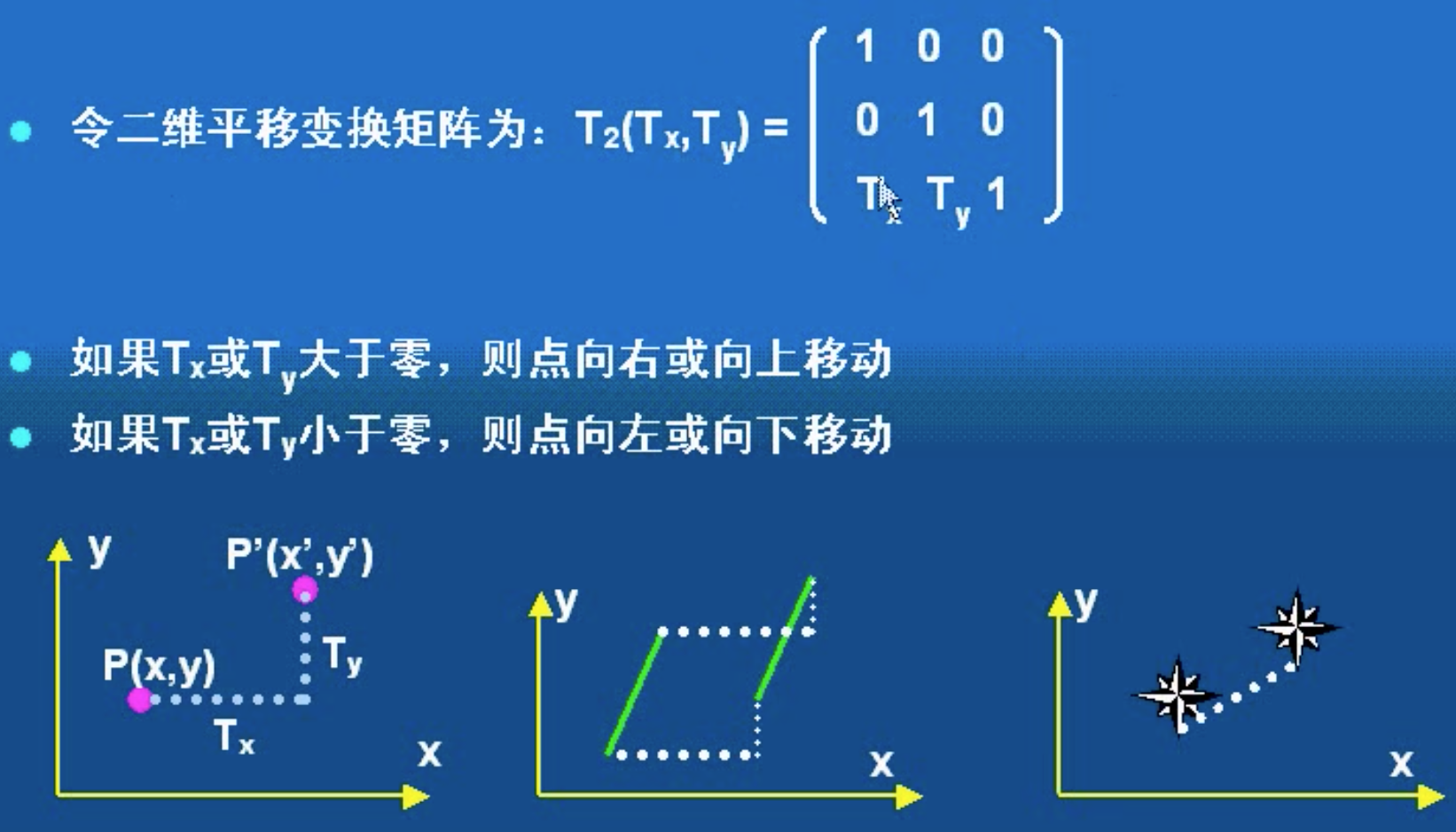三、缩放变换

点的缩放是指该点沿x轴和y轴方向按比例缩小或放大的变换。设图形上的点P(x,y),在X轴和Y轴方向分表作Sx和Sy的缩放，结果生成新的点坐标P‘(x',y'),如同说是，则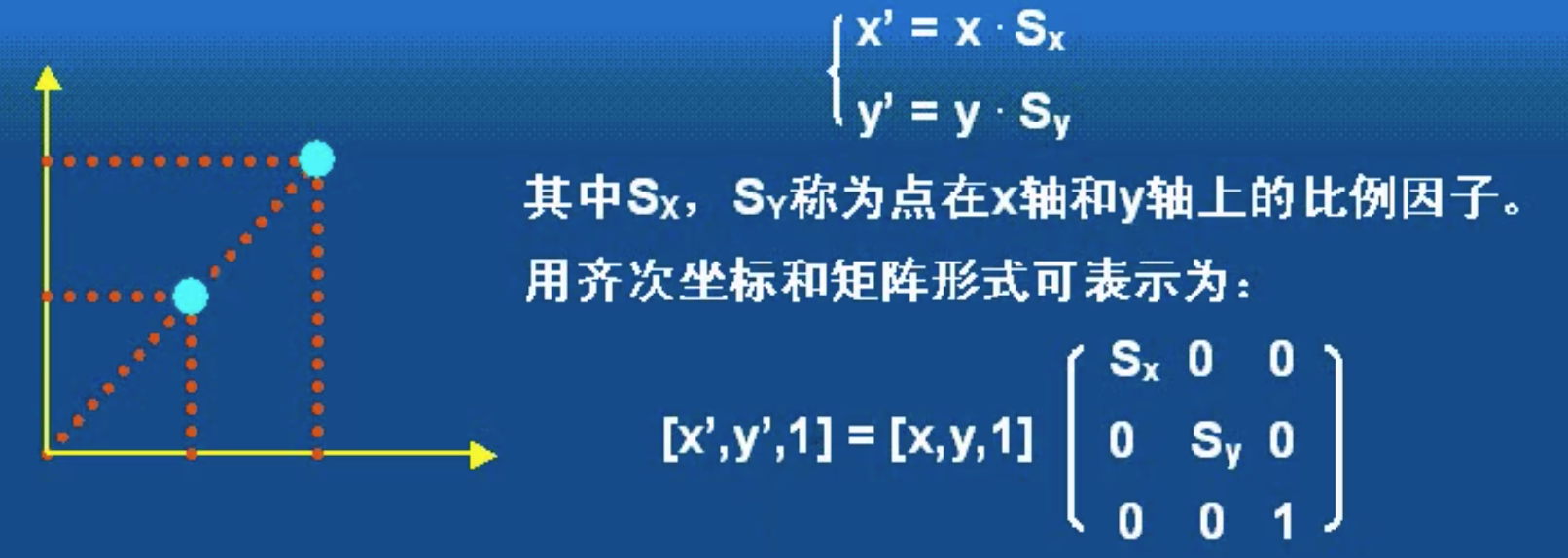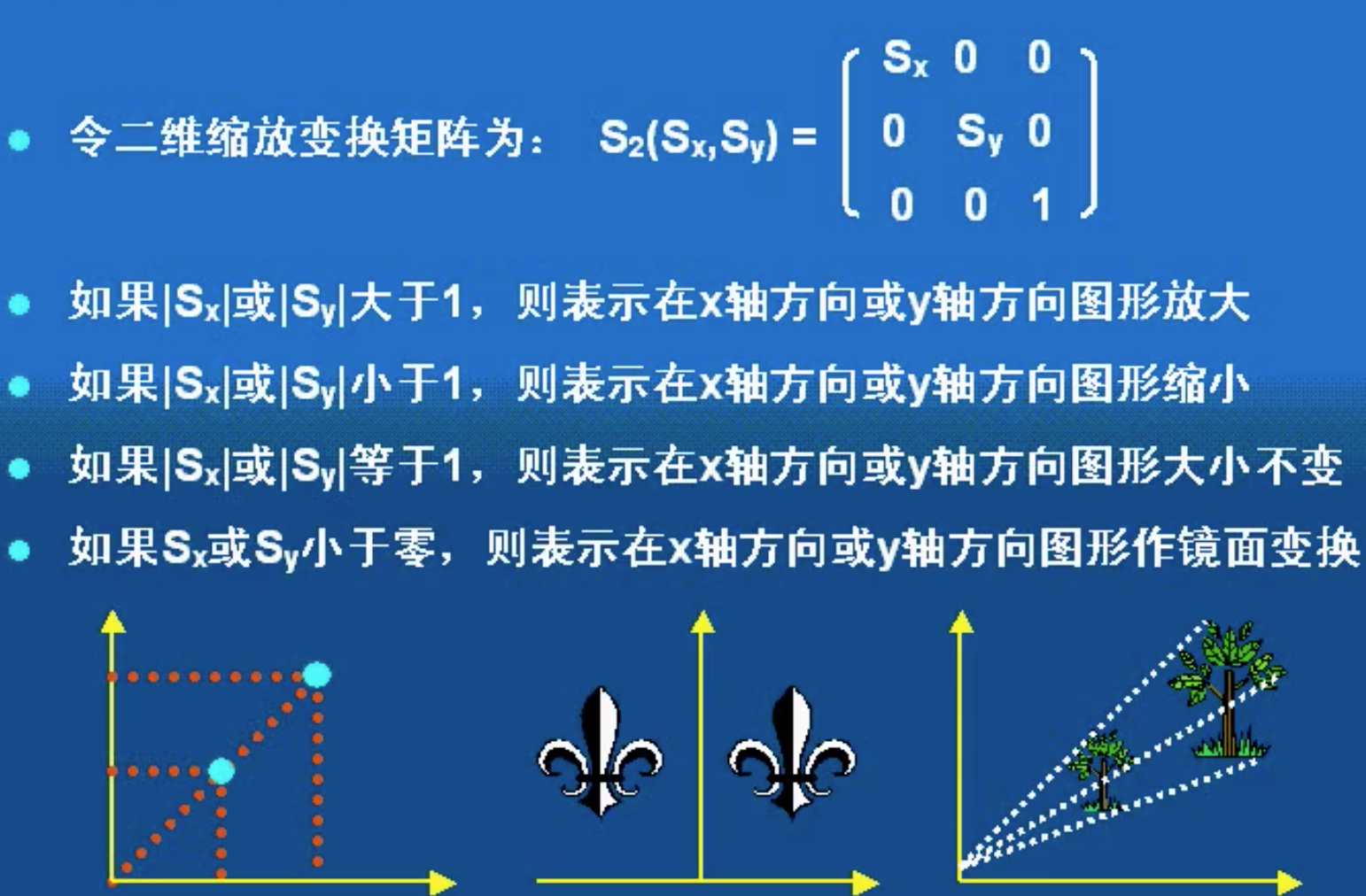四、旋转变换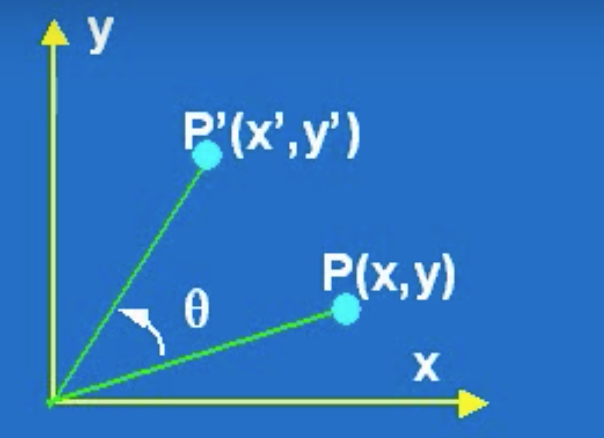点的旋转变换是指将点绕坐标远点旋转一角度的坐标变换。设有图形上点P(x,y),将器绕原点旋转变换 θ角度(假设按逆时针旋转为正角)，结果生成的新的点坐标P‘(x',y')

将点P绕原点做逆时针旋转θ角度的变换看作将坐标系原点做顺时针旋转θ角度的等价变换

x' = xcosθ-ysinθ

y' = xsinθ+ycosθ

其中θ为点绕原点旋转的角度(逆时针为正，顺时针为负)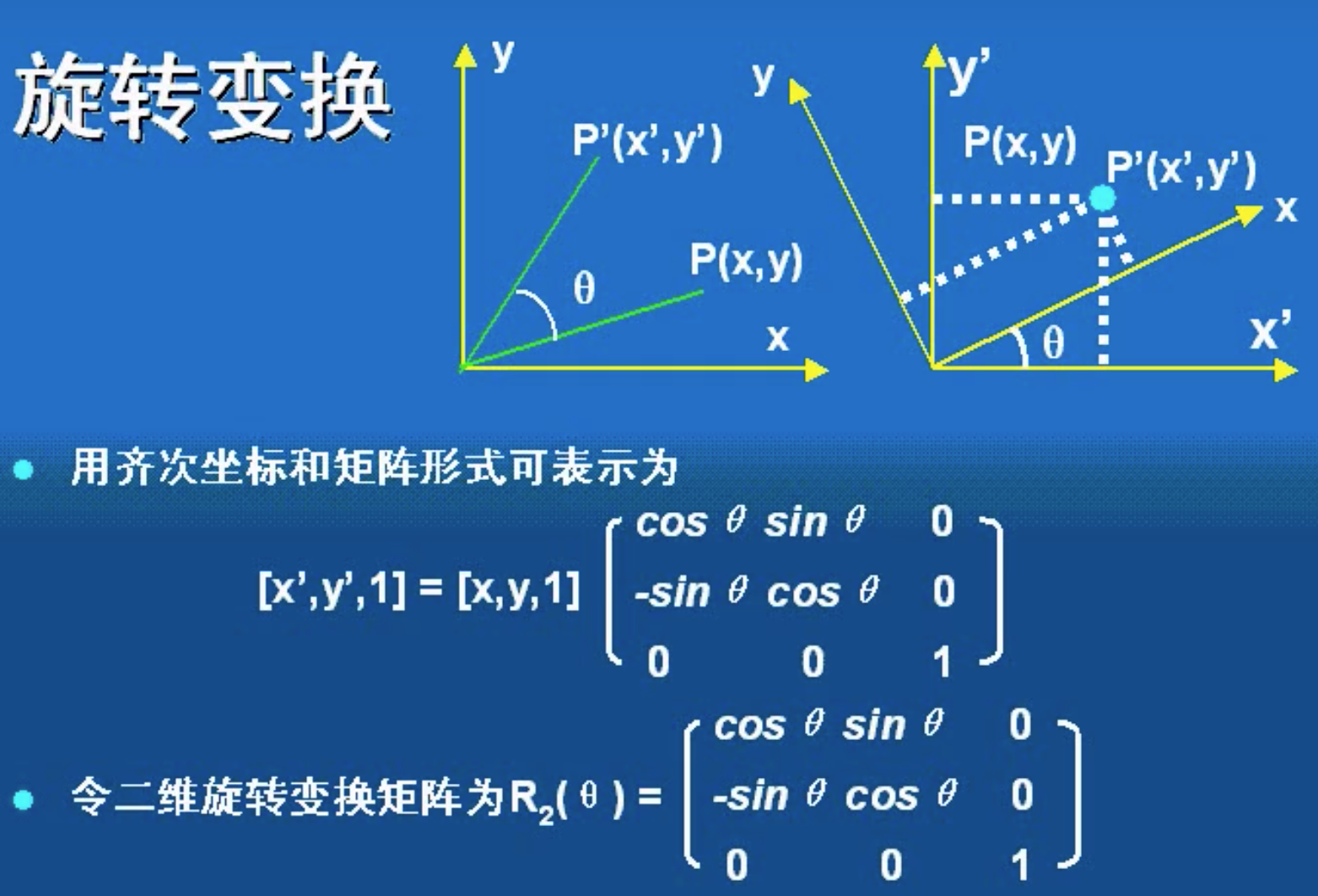五、变形变换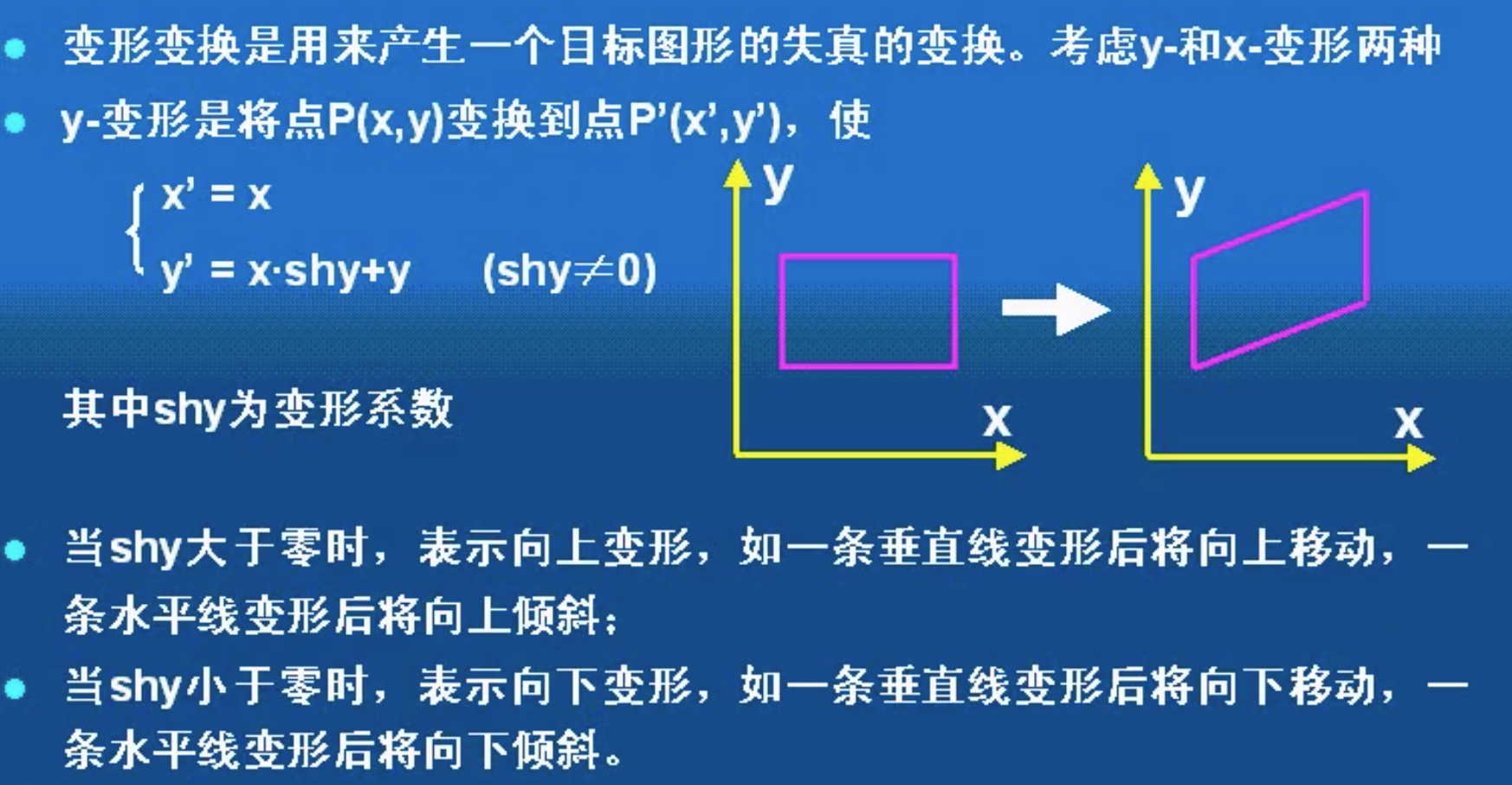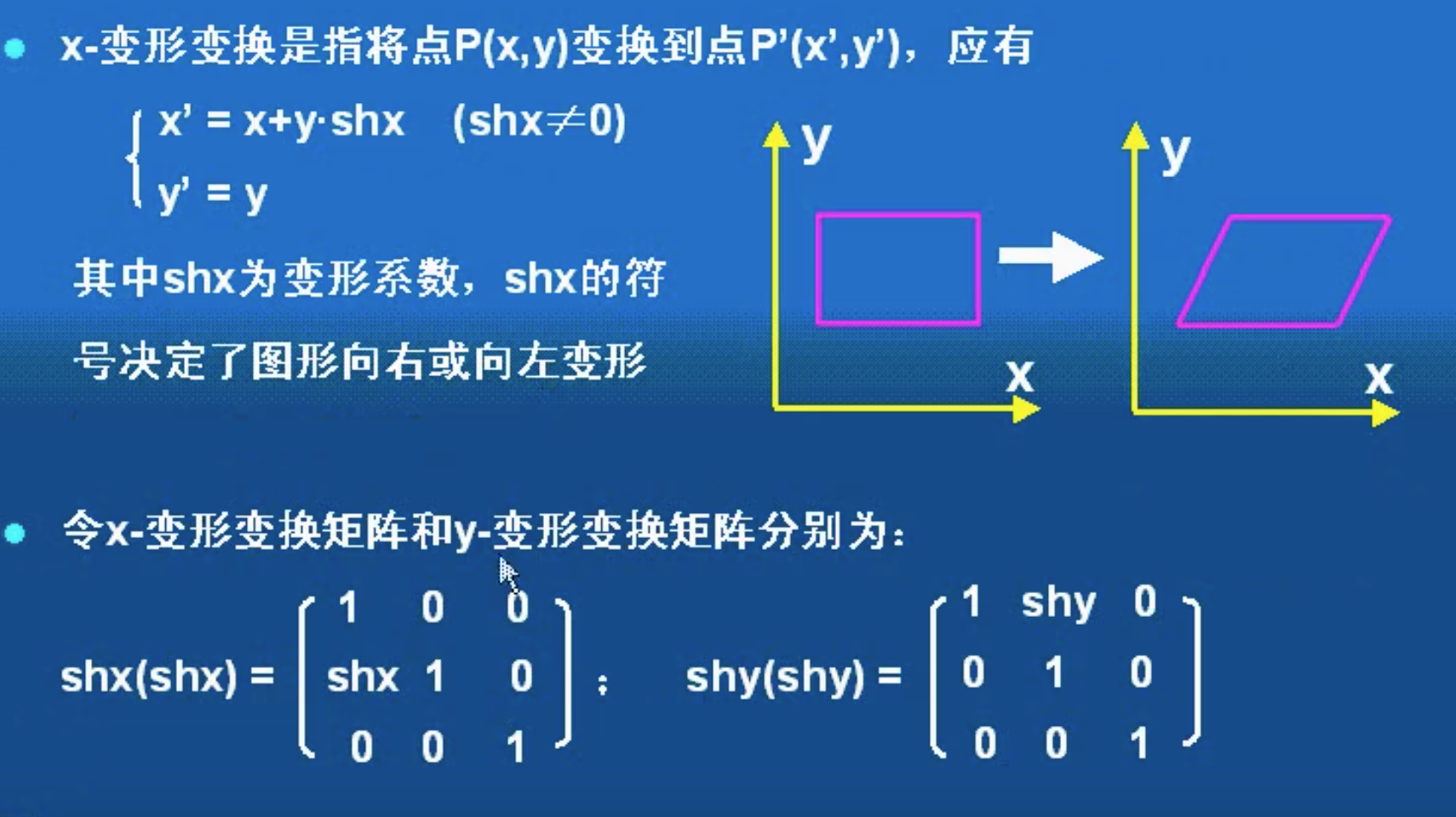六、组合变换

实际上，一般的图形变换更多的是组合变换，即有一系列基本的几何变换结合而成的，则组合变换矩阵也可由一系列基本几何变换举证的乘积来表示，矩阵的乘法满足结合律，但不满足交换律。


展开全文• 文章目录一.缩放1.原理2.函数调用二.翻转三.仿射1.实现图像平移2.旋转3.实现更复杂的仿射四.透视五....一....在缩放的过程实际是改变图像的尺寸，那么他们的像素点的位置也就发生了变化，再缩放过程中最核心的两步： ...
• 这次学习图像的几何变换，主要有以下内容：　·图像的平移变换　·图像的镜像变换　·图像的转置变换　·图像的旋转变换　·图像的缩放 1、图像的平移变换　在进行书写matlab代码之前，先来了解一下图像平移的理论...
• ## 图像的几何变换

万次阅读 2016-12-22 14:38:57
包含相同内容的两幅图像可能由于成像角度、透视关系乃至镜头自身原因所造成的几何失 真而呈现出截然不同的外观，这就给观测者或是图像识别程序带来了...因此， 几何变换常常作为其他图像处理应用的预处理步骤， 是图图像处理 应用
• 几何变换 几何变换可以看成图像中物体（或像素）空间位置改变，或者说是像素的移动。 几何运算需要空间变换和灰度级差值两个步骤的算法，像素通过变换映射到新的坐标位置，新的位置可能是在几个像素之间，即不一定...float matrix 图像处理
• ## 二维图形基本几何变换矩阵

万次阅读 多人点赞 2018-06-18 21:12:49
二维图形基本几何变换是指相对于坐标原点和坐标轴进行的几何变换，包括平移（Translate）、比例（Scale）、旋转（Rotate）、反射（Reflect）和错切（shear）5种变换。物体变换物体变换是通过变换物体上每一个顶点...
• 1、基本几何变换及变换矩阵 基本几何变换都是相对于坐标原点和坐标轴进行的几何变换，有平移、比例、旋转、反射和错切等。 1.1 平移变换 是指将p点沿直线路径从一个坐标位置移到另一个坐标位置的重定位过程。他是...
• 几何图形变换这部分内容很简单。 其中主要我这里主要包括2种图形的变换。 1.直线 2.多边形 实现的变换类型是4种 1.平移 平移是指对一个图形移到另外一个位置即： 通过将位移量加到一个点的坐标上来生成一个新的点。 ...二维图形几何变换 计算机图形学 旋转变换 几何变换
• 继之前的http://blog.csdn.net/piaoxuezhong/article/details/62430051绕轴旋转，这里...基本三维几何变换 1. 平移变换 若空间平移量为(tx, ty, tz)，则平移变换为   2. 比例变换 （1） 相对坐标原点的比例变换矩阵变换
• 图形变换：一般是指对图形的几何信息经过变换后产生新的图形。 图形变换的实质：改变图形的坐标位置。一个图形的基本要素是点，点构成线，线构成面，若干面构成体，因此只要改变了图形上的各点坐标位置，整个图形...
• 1 平移 所有像素加上或减去指定的水平或垂直偏移量 I=imread('songshu.jpg'); I=double(I); B=zeros(size(I));...B(move_y+1:H(1),move_x+1:H(2),1:H(3))=I(1:H(1)-move_y,1:H(2)-move_x,1:H(3));...matlab
• 几何图像按照某种法则或规律变换成另一种几何图像的过程叫做图像的几何变换。图像的几何变换不改变图像像素值，只是将一幅图像中的位置坐标映射到另一幅图像中。常见的几何变换有平移、镜像、旋转、缩放、仿射等。...
• 什么是正交变换？正交变换为何要满足下列条件？正交变换研究什么？ 1 表象 2 正交变换：研究”长度不变“ 3 性质 角度，长度，面积不变 4 基本形式 （1）平移变换 （2）旋转变换 （3）轴反射变换 ...高等几何 三维重建
• 明白以下问题 ... 几何是研究各种变换群下的不变量。工程上常用的几何是 1）拓扑：对应的变换群是拓扑同胚，比如把一个橡皮环，只要不拉破，他的拓扑是不变的 2）黎曼几何：等距变换；这里的距是指测地距离。把
• 1 几何畸变 遥感成像过程中，传感器生成的图像像元相对于地面目标物的实际位置发生了挤压、拉伸、扭曲和偏移等问题。 几何畸变产生的原因：1）传感器内部因素；2）遥感平台因素；3）地球因素。 因为存在几何畸变...几何校正
• ## 矩阵乘法的几何意义

万次阅读 热门讨论 2018-04-15 20:30:41
最近开始复习线代线代真的该画画图不要只是背公式算可能太高维的不容易想象 但是低维画画图能加深理解对了 要将矩阵看作变换2*3矩阵a 1 -1 20 2 -1三个基向量即图中蓝线俯视（1,0）（-1,2）（2,-1）分别是矩阵a...
...